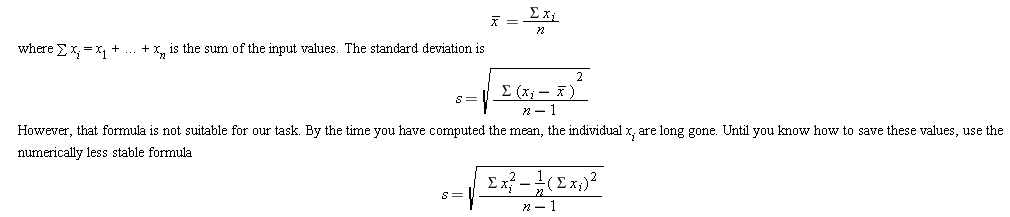Mean and standard deviation. Write a program that reads a set of floating-point data values from the input. When the user indicates the end of input, print out the count of the values, the average, and the standard deviation. The average of a data set x1,…,xn isYou can compute this quantity by keeping track of the count, the sum, and the sum of squares in the DataSet class as you process the input values.

Complete the following files:

### DataSet.java

Use the following file:

DataSetTester.java

```import java.util.Scanner;

/**
This is a test driver class for DataSet class.
*/
public class DataSetTester
{
public static void main(String[] args)
{
DataSet a = new DataSet();